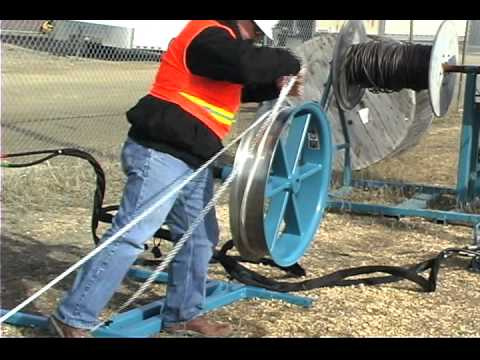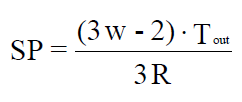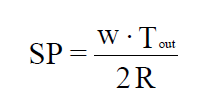# Different Cable Pulling parametersThe pulling parameters of the cable include the maximum pulling stress and the side wall pressure calculations. The voltage calculations take into account the maximum permissible voltage that the traction device and the conductors can withstand.

The calculation of the side wall pressure takes into account the stress effects that a curve in the conduit or cable tray has on the cable during the extraction operation.

The general procedure used to determine the tensions and tensile parameters of the cable is to calculate the tensile stresses for the entire length of the traction and then determine if the pressure of the side wall is too great in the curves of the conduit.

This section of the Module describes cable pulling parameters in terms of the following topics:

• Maximum Pulling Tension
• Sidewall Pressure
• Rigging Method Effects Calculations

## Maximum Pulling Tensions:

Once the size of the raceway has been determined (according to the allowable fill, jam ratio and free space of the cable), the maximum tension for the pulling device and the maximum tension that can be applied must be calculated. safely to the drivers.

he most limited maximum voltage is used as the maximum allowable tensile stress ™. Next, the tensile stress (T) that is actually required to pass the cable through the raceway is calculated and compared with the maximum tensile stress allowed

When the actual tensile stress exceeds the maximum tensile stress allowed for the installation, the following actions should be considered:

• Increase the radius of curvature that will be used for conduit installation.

• Decrease the permuted filling of ducts.

• Reduce the number of bends in the conduit.

• Perform a reverse pull.

• Perform the pull in stages.

• Decrease the length of the pull.

The maximum pulling tension that can be applied to a single-conductor (copper conductor) cable is calculated through use of the following equation:

## Sidewall Pressure:

The radius of curvature of a conduit or cable tray is limited by two factors:

The minimum training radius of the cable under static load and the pressure of the side wall that will be exerted on the cable during its installation. The sidewall pressure (SP) is defined as the crushing force (in Newtons or pounds-force) exerted on a cable when it is pulled around a bent section of the conduit or conduit.

SP is directly proportional to the tensile stress that leaves the curve and is inversely proportional to the radius of the curve; that is, as the tensile stress increases, the side wall pressure also increases, and as the bending radius decreases, SP increases.

The equation that is used to calculate the SP that is exerted on a cable depends on the configuration of the cables that are in the conduit. The equations are the following:

• For a single conductor installation, the following equation should be used to calculate the SP:

SP = Tout / R

• For three cables that are in a cradled configuration, the following equation should be used:• For three cables that are in a triangular configuration, the following equation should be used:Once the tensile stresses have been evaluated along the entire raceway, the SPs are calculated for each curve and compared with the maximum limit of SP allowed. If the SP of any curve exceeds the allowed limit, the radius of that curve must be increased.